# JP6575742B2 - Image processing method and image processing apparatus - Google Patents

## Info

Publication number
JP6575742B2
JP6575742B2 JP2015012814A JP2015012814A JP6575742B2 JP 6575742 B2 JP6575742 B2 JP 6575742B2 JP 2015012814 A JP2015012814 A JP 2015012814A JP 2015012814 A JP2015012814 A JP 2015012814A JP 6575742 B2 JP6575742 B2 JP 6575742B2
Authority
JP
Japan
Prior art keywords
pixel
image
coefficient
pixel value
plurality
Prior art date
Legal status (The legal status is an assumption and is not a legal conclusion. Google has not performed a legal analysis and makes no representation as to the accuracy of the status listed.)
Active
Application number
JP2015012814A
Other languages
Japanese (ja)
Other versions
JP2015179500A (en
Inventor

Original Assignee
パナソニックＩｐマネジメント株式会社
Priority date (The priority date is an assumption and is not a legal conclusion. Google has not performed a legal analysis and makes no representation as to the accuracy of the date listed.)
Filing date
Publication date
Priority to JP2014035000 priority Critical
Priority to JP2014035000 priority
Application filed by パナソニックＩｐマネジメント株式会社 filed Critical パナソニックＩｐマネジメント株式会社
Priority to JP2015012814A priority patent/JP6575742B2/en
Publication of JP2015179500A publication Critical patent/JP2015179500A/en
Application granted granted Critical
Publication of JP6575742B2 publication Critical patent/JP6575742B2/en
Application status is Active legal-status Critical
Anticipated expiration legal-status Critical

• 238000003672 processing method Methods 0 claims description title 18
• 230000002829 reduced Effects 0 claims description 101
• 230000000875 corresponding Effects 0 claims description 85
• 230000000670 limiting Effects 0 claims description 37
• 238000000034 methods Methods 0 claims description 32
• 230000001603 reducing Effects 0 claims description 27
• 238000006722 reduction reaction Methods 0 description 19
• 238000003702 image correction Methods 0 description 15
• 230000000007 visual effect Effects 0 description 10
• 230000015556 catabolic process Effects 0 description 8
• 238000006731 degradation Methods 0 description 8
• 230000004059 degradation Effects 0 description 8
• 230000004048 modification Effects 0 description 8
• 238000006011 modification Methods 0 description 8
• 230000001629 suppression Effects 0 description 7
• 230000007274 generation of a signal involved in cell-cell signaling Effects 0 description 6
• 230000000694 effects Effects 0 description 4
• 239000002609 media Substances 0 description 3
• 230000015654 memory Effects 0 description 3
• 239000011159 matrix materials Substances 0 description 2
• 230000000576 supplementary Effects 0 description 2
• 241000282412 Homo Species 0 description 1
• 241000282414 Homo sapiens Species 0 description 1
• 238000007792 addition Methods 0 description 1
• 238000005282 brightening Methods 0 description 1
• 238000004364 calculation methods Methods 0 description 1
• 238000006243 chemical reaction Methods 0 description 1
• 238000005516 engineering processes Methods 0 description 1
• 230000004438 eyesight Effects 0 description 1
• 230000014509 gene expression Effects 0 description 1
• 230000001965 increased Effects 0 description 1
• 239000004973 liquid crystal related substances Substances 0 description 1
• 238000005070 sampling Methods 0 description 1

## Images

•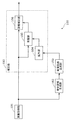•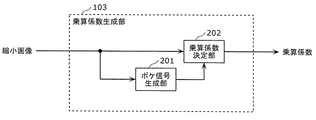••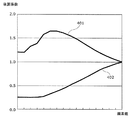•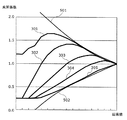•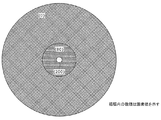•••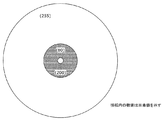•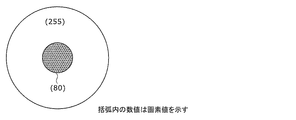•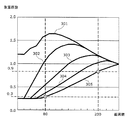•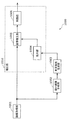•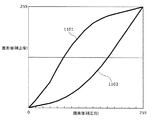•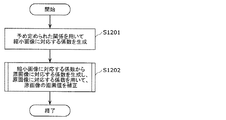•## Classifications

• GPHYSICS
• G06COMPUTING; CALCULATING; COUNTING
• G06TIMAGE DATA PROCESSING OR GENERATION, IN GENERAL
• G06T5/00Image enhancement or restoration
• G06T5/007Dynamic range modification

## Description

The present disclosure relates to an image processing method for correcting the brightness of an original image for each pixel.

Patent Document 1 discloses a visual processing device. In this visual processing device, a first gain signal having a predetermined gain characteristic with respect to the image signal is output by the visual processing unit, and the image signal input to the visual processing device is corrected based on the first gain signal. . Therefore, high-performance correction processing can be realized with a simple configuration.

JP 2007-31349 A

The present disclosure provides an image processing method capable of satisfying both a reduction in processing amount required for generating a coefficient for correcting an original image and suppression of image quality degradation caused by the reduction in processing amount.

An image processing method according to the present disclosure is an image processing method for correcting the brightness of an original image composed of a plurality of pixels each having a pixel value related to brightness for each pixel, the number of pixels of the original image Reduced image generation step for generating a reduced image of the original image by reducing the pixel value of the processing target pixel and the pixel of the processing target pixel for each brightness state related to the brightness around the processing target pixel by using a predetermined relationship to a coefficient for correcting the value, before Kichijimi a coefficient generation step of generating coefficients for each pixel a respective plurality of pixels of the small image as the processing target pixel, wherein the coefficient for each pixel of said original image from the coefficients of each pixel of the reduced image form raw, and a correction step of correcting the pixel value of each pixel of said original image by using a coefficient for each pixel of said original image, In the correction step, A process of limiting the coefficient based on a predetermined relationship in generating the coefficient for each pixel of the coefficients or found before Kihara image for each pixel of the serial reduced image, the original image based on said predetermined relationship At least one of the processes for limiting the pixel value after correction of the pixel.

The image processing method according to the present disclosure can achieve both reduction in the amount of processing required to generate a coefficient for correcting an original image and suppression of image quality degradation caused by the reduction in the amount of processing.

FIG. 1 is a configuration diagram illustrating a specific example of the image processing apparatus according to the first embodiment. FIG. 2 is a configuration diagram illustrating a specific example of the multiplication coefficient generation unit according to the first embodiment. FIG. 3 is a diagram illustrating a specific example of the two-dimensional LUT according to the first embodiment. FIG. 4 is a diagram illustrating an example of a limited range in the first embodiment. FIG. 5 is a diagram illustrating an example of a limited range in the first embodiment. FIG. 6A is a diagram illustrating an example of a pixel value of an original image in the first embodiment. FIG. 6B is a diagram illustrating an example of pixel values of the reduced image according to Embodiment 1. FIG. 7 is a diagram illustrating an example of limited multiplication coefficients in the first embodiment. FIG. 8A is a diagram showing an example of the pixel value of the original image in the first embodiment. FIG. 8B is a diagram illustrating an example of the pixel value of the reduced image in the first embodiment. FIG. 9 is a diagram illustrating an example of a limited multiplication coefficient in the first embodiment. FIG. 10 is a configuration diagram illustrating a specific example of the image processing apparatus according to the second embodiment. FIG. 11 is a diagram illustrating an example of a limited range in the second embodiment. FIG. 12 is a flowchart showing the operation of the image processing apparatus according to the first or second embodiment. FIG. 13 is a flowchart illustrating the operation of the correction unit according to the first or second embodiment.

Hereinafter, embodiments will be described in detail with reference to the drawings as appropriate. However, more detailed description than necessary may be omitted. For example, detailed descriptions of already well-known matters and repeated descriptions for substantially the same configuration may be omitted. This is to avoid the following description from becoming unnecessarily redundant and to facilitate understanding by those skilled in the art.

The inventor provides the accompanying drawings and the following description in order for those skilled in the art to fully understand the present disclosure, and is not intended to limit the subject matter described in the claims. Absent.

(Embodiment 1)
Hereinafter, the present embodiment will be described with reference to FIGS.

[1-1. Constitution]
FIG. 1 is a configuration diagram illustrating a specific example of an image processing apparatus according to the present embodiment. The image processing apparatus 100 illustrated in FIG. 1 includes an image acquisition unit 101, a reduced image generation unit 102, a multiplication coefficient generation unit 103, an enlargement unit 104, a restriction unit 105, and an image correction unit 106. The enlargement unit 104, the restriction unit 105, and the image correction unit 106 constitute a correction unit 110.

The image acquisition unit 101 acquires an image as an original image. For example, the image acquisition unit 101 acquires an original image from a recording medium connected to the image processing apparatus 100. The original image may be a picture included in a moving image stream or a still image. Hereinafter, for convenience of description, a picture included in a moving image stream will be described as an original image to be processed.

Specifically, the image acquisition unit 101 acquires one picture at an arbitrary time included in the input moving image stream as an original image. Note that the video stream decoding process may be executed by the image processing apparatus 100 or may be executed by an external apparatus. In the latter case, the image acquisition unit 101 acquires a decoded moving image stream.

Here, the image is composed of a plurality of pixels. The pixel has a pixel value related to the brightness of the pixel. For example, the brighter the pixel, the larger the pixel value of the pixel, and the darker the pixel, the smaller the pixel value of the pixel. Therefore, a large pixel value may be expressed as a bright pixel value, and a small pixel value may be expressed as a dark pixel value. The pixel value may be a luminance value, an RGB value component, or a YUV value component.

The reduced image generation unit 102 reduces the image. For example, the reduced image generation unit 102 reduces the number of pixels of the original image acquired by the image acquisition unit 101 and generates a reduced image.

Specifically, the reduced image generation unit 102 generates a reduced image without aliasing distortion by performing low-pass filter processing (low-pass filter processing) and sub-sampling processing. For example, when the original image is 1920 × 1080 pixels, the reduced image generation unit 102 reduces the original image to an image having a pixel number of 1/4 each in the horizontal and vertical directions, thereby reducing the reduced image having 480 × 270 pixels. Is generated.

Note that when the reduced image generation unit 102 generates a reduced image, the reduced image generation unit 102 may generate a reduced image having the same aspect ratio as the original image, or may generate a reduced image having another aspect ratio. . That is, the designer can freely set the number of pixels and the aspect ratio of the reduced image.

Then, the reduced image generation unit 102 outputs a reduced image obtained by reducing the original image to the multiplication coefficient generation unit 103.

In the above description, filter processing has been described as a method for generating a reduced image. However, the reduced image generation unit 102 calculates (calculates) an average value of a plurality of pixel values of the original image for one pixel after reduction. The average value may be generated as the pixel value of one pixel of the reduced image.

The multiplication coefficient generation unit 103 is a generation unit that generates a multiplication coefficient. Specifically, the multiplication coefficient generation unit 103 calculates, for each pixel of the reduced image, a multiplication coefficient based on the pixel value of the pixel of the reduced image and the pixel value of pixels located around the pixel. Thus, a plurality of multiplication coefficients corresponding to a plurality of pixels of the reduced image are generated. More specifically, the multiplication coefficient generation unit 103 generates each multiplication coefficient based on the characteristic information of the multiplication coefficient that changes depending on the brightness state (brightness / darkness state) related to the brightness around the processing target pixel.

For example, the characteristic information indicates the relationship between the pixel value of the processing target pixel, the brightness state related to the brightness around the processing target pixel, and the multiplication coefficient for correcting the pixel value of the processing target pixel. Specifically, the brightness state is the brightness at a plurality of pixels including the processing target pixel and the periphery of the processing target pixel.

The multiplication coefficient generated by the multiplication coefficient generation unit 103 is a coefficient for correcting the pixel value of the pixel corresponding to the pixel of the reduced image among the plurality of pixels of the original image. This multiplication coefficient may be regarded as a coefficient for correcting the pixel value of the pixel of the reduced image. In addition, this multiplication coefficient may be expressed as a gain. In addition, a plurality of multiplication coefficients each corresponding to an image pixel may correspond to an image and may be expressed as a gain map. Such a plurality of multiplication coefficients can also be expressed as a coefficient map, a coefficient matrix, a coefficient matrix, or coefficient data.

Further, as described above, this multiplication coefficient is generated for each pixel included in the reduced image. Then, the same number of multiplication coefficients as the number of pixels of the reduced image are expanded in a later process, and the same number of multiplication coefficients as the number of pixels of the original image are generated.

FIG. 2 is a configuration diagram illustrating a specific example of the multiplication coefficient generation unit 103 illustrated in FIG. 1, for explaining a specific example of generating a multiplication coefficient from a reduced image input to the multiplication coefficient generation unit 103. FIG. For example, as illustrated in FIG. 2, the multiplication coefficient generation unit 103 includes a blur signal generation unit 201 and a multiplication coefficient determination unit 202.

For each pixel of the reduced image, the blur signal generation unit 201 combines the pixel value of the pixel and the pixel values of a plurality of pixels located around the pixel to generate a blur signal. The blur signal includes information indicating the brightness of the target pixel and the surrounding area (that is, the average brightness of the target pixel and the surrounding area).

Here, the periphery is, for example, a range of one pixel or a predetermined number of pixels. The plurality of pixels located around the target pixel may be a plurality of pixels adjacent to the target pixel. Here, the pixel value of the target pixel is used to generate the blur signal, but only the pixel value of the pixels located around the target pixel may be used. Further, the position of the pixel may correspond to the center of the pixel, or may correspond to the upper left corner of the pixel.

Specifically, the blur signal generation unit 201 uses a two-dimensional low-pass filter, a one-dimensional low-pass filter, or the like to filter the pixel values (for example, luminance values) of the target pixel and the surrounding pixels. Process. Then, the blur signal generation unit 201 outputs the blur signal obtained by the filter processing to the multiplication coefficient determination unit 202.

The multiplication coefficient determining unit 202 determines the multiplication coefficient for each pixel of the reduced image based on the pixel value of the pixel and the blur signal corresponding to the pixel, thereby making the number of pixels the same as the number of pixels of the reduced image. Generate a multiplication factor. For example, if the reduced image has 480 × 270 pixels, 480 × 270 multiplication coefficients are generated.

Specifically, the multiplication coefficient determination unit 202 generates a multiplication coefficient from the pixel value of the reduced image and the blur signal using a two-dimensional LUT (two-dimensional lookup table). Here, the two-dimensional LUT is a table for determining the multiplication coefficient based on the pixel value of the reduced image and the blur signal. That is, in the two-dimensional LUT, the multiplication coefficient corresponding to the pixel value of the reduced image and the blur signal is determined.

For example, the two-dimensional LUT includes a plurality of tables each indicating a correspondence relationship between a plurality of pixel values and a plurality of multiplication coefficients. The multiplication coefficient determination unit 202 selects a table from among a plurality of tables using the blur signal input from the blur signal generation unit 201. Then, the multiplication coefficient determination unit 202 generates a multiplication coefficient by determining the multiplication coefficient corresponding to the pixel value of the reduced image with reference to the selected table. Note that a function for determining the multiplication coefficient based on the pixel value of the reduced image and the blur signal may be used in the two-dimensional LUT.

Further, for example, the multiplication coefficient determination unit 202 generates a multiplication coefficient for dark part correction. The dark part correction is a correction that brightens a dark area (dark part) and is a correction that corresponds to human visual characteristics. Such dark portion correction may be combined with bright portion correction that darkens a bright region (bright portion).

Humans have visual characteristics that unconsciously transform information that enters the eye. In the two-dimensional LUT used in the multiplication coefficient determination unit 202, a conversion characteristic that imitates a visual characteristic called light / dark contrast or color contrast is simply expressed as characteristic information. Specifically, even if the brightness is physically the same, the same brightness is perceived as dark when the surroundings are bright, and the same brightness is perceived as bright when the surroundings are dark. Such visual characteristics are called light-dark contrast.

The multiplication coefficient determination unit 202 generates a multiplication coefficient corresponding to such visual characteristics in order to improve subjective image quality. In particular, the multiplication coefficient determination unit 202 generates a multiplication coefficient using a blur signal that reflects surrounding pixel values in order to suppress the disappearance of contrast due to correction. Thereby, an appropriate multiplication coefficient is generated and the disappearance of contrast is suppressed.

FIG. 3 is a diagram illustrating an example of a two-dimensional LUT used in the multiplication coefficient determination unit 202. In FIG. 3, the horizontal axis represents the pixel value of the pixel to be processed, and the vertical axis represents the multiplication coefficient. In the following description, the range of the pixel value of the processing target pixel and the value of the blur signal (blur signal value) is assumed to be 0 to 255.

The multiplication coefficient determination unit 202 selects a one-dimensional characteristic table according to the blur signal value. Specifically, when the blur signal value is 0, the multiplication coefficient determination unit 202 selects the one-dimensional characteristic table 301.

Similarly, when the blur signal value is 64, the multiplication coefficient determination unit 202 selects the one-dimensional characteristic table 302. When the blur signal value is 128, the multiplication coefficient determination unit 202 selects the one-dimensional characteristic table 303. When the blur signal value is 196, the multiplication coefficient determination unit 202 selects the one-dimensional characteristic table 304. When the blur signal value is 255, the multiplication coefficient determination unit 202 selects the one-dimensional characteristic table 305.

In FIG. 3, for the sake of convenience, a one-dimensional characteristic table in which the blur signal values are 0, 64, 128, 196, and 255 is described, but the two-dimensional LUT has a different shape for each blur signal value. It has a dimension characteristic table. The multiplication coefficient determination unit 202 selects a one-dimensional characteristic table according to the blur signal value. Then, the multiplication coefficient determination unit 202 calculates the multiplication coefficient corresponding to the pixel value of the processing target pixel as the multiplication coefficient corresponding to the processing target pixel in the selected one-dimensional characteristic table.

For example, when the blur signal value is 128 and the pixel value is 80, the multiplication coefficient determination unit 202 processes the multiplication coefficient 0.6 corresponding to the point 306 on the one-dimensional characteristic table 303 in FIG. Calculated as a multiplication coefficient corresponding to the target pixel.

Ideally, the two-dimensional LUT indicates all multiplication coefficients for (the number of gradations of the blur signal value) × (the number of gradations of the pixel value of the reduced image). However, a plurality of multiplication coefficients indicated by the two-dimensional LUT may be discretized and expressed by a broken line for simplification. In that case, when the multiplication coefficient determination unit 202 refers to the multiplication coefficient between the plurality of discretized multiplication coefficients, the multiplication coefficient is interpolated by interpolating the multiplication coefficient between the plurality of multiplication coefficients from the plurality of multiplication coefficients. Refers to the multiplication factor.

The enlargement unit 104 generates a plurality of multiplication coefficients corresponding to the plurality of pixels of the original image from a plurality of multiplication coefficients corresponding to the plurality of pixels of the reduced image, thereby generating a plurality of pixels corresponding to the plurality of pixels of the reduced image. The multiplication coefficient is expanded to a plurality of multiplication coefficients corresponding to a plurality of pixels of the original image. Here, the expansion of the plurality of multiplication coefficients corresponds to increasing the number of multiplication coefficients instead of changing each multiplication coefficient to a large coefficient. Therefore, the expansion may be expressed as expansion.

In other words, the enlargement unit 104 generates the gain map of the original image from the gain map of the reduced image, thereby expanding the gain map of the reduced image to the gain map of the original image. Furthermore, in other words, the enlargement unit 104 expands a plurality of multiplication coefficients corresponding to a plurality of pixels of the reduced image into a plurality of multiplication coefficients corresponding to a plurality of pixels of the original image.

Specifically, for example, the enlargement unit 104 enlarges a plurality of multiplication coefficients by an enlargement process using bilinear interpolation (linear interpolation). As another method, the enlargement unit 104 enlarges a plurality of multiplication coefficients by an enlargement process using nearest neighbor interpolation. As another method, the enlargement unit 104 enlarges a plurality of multiplication coefficients by an enlargement process using bicubic interpolation.

The restriction unit 105 restricts the multiplication coefficient generated for each pixel included in the original image with an upper limit, a lower limit, or both according to the pixel value of the pixel. Details of the restriction unit 105 will be described later.

The image correction unit 106 corrects the original image. For example, the image correction unit 106 performs dark portion correction or bright portion correction on the original image. Specifically, the image correction unit 106 corrects the pixel value of the pixel by multiplying the pixel value of the pixel by a multiplication coefficient generated for each pixel included in the original image.

For example, the image correction unit 106 generates a pixel value (X out ) obtained by multiplying the pixel value (X in ) of the original image by the multiplication coefficient (g) as shown in Equation 1. Then, the image correction unit 106 corrects the original image by generating an image composed of the generated pixel values (X out ).

X out = gX in (Expression 1)

More specifically, the image correction unit 106 performs correction processing on each component of the RGB value as the pixel value of the original image. As another method, the image correction unit 106 may perform correction processing on each component of the YUV value as the pixel value of the original image.

[1-2. Details of Restriction Unit 105]
Hereinafter, specific operations in the limiting unit 105 will be described with reference to the drawings.

The restriction unit 105 calculates a restriction value for the multiplication coefficient according to the pixel value of the target pixel in the original image. Specifically, the limit unit 105 calculates a limit value using a one-dimensional characteristic table obtained for the pixel value of the target pixel from the two-dimensional LUT used by the multiplication coefficient generation unit 103. Then, the limiting unit 105 limits the multiplication coefficient with the calculated limit value. In short, the limiting unit 105 limits the multiplication coefficient corresponding to the pixel of the original image within the range set by the pixel value of the original image and the characteristic information used by the multiplication coefficient generation unit 103.

FIG. 4 is a diagram illustrating an example of a limited range in the present embodiment. FIG. 4 shows a one-dimensional characteristic table 401 for upper limit restriction and a one-dimensional characteristic table 402 for lower limit restriction.

The restriction unit 105 creates a one-dimensional characteristic table by projecting the two-dimensional LUT used by the multiplication coefficient generation unit 103 to one dimension. For example, the upper limit limiting one-dimensional characteristic table 401 includes a plurality of multiplications obtained from a plurality of blur signal values from 0 to 255 for each pixel value from 0 to 255 in the two-dimensional LUT shown in FIG. It consists of the largest multiplication coefficient among the coefficients. Similarly, the one-dimensional characteristic table 402 for lower limit restriction is configured with the smallest multiplication coefficient for each pixel value of 0 to 255.

In short, the limiting unit 105 sets the maximum multiplication coefficient among the plurality of multiplication coefficients calculated corresponding to the plurality of blur signal values for each pixel value using the characteristic information of the multiplication coefficient as the upper limit of the multiplication coefficient for the pixel value. Set as. Further, the limiting unit 105 uses the characteristic information of the multiplication coefficient to calculate the minimum multiplication coefficient among the plurality of multiplication coefficients calculated for each pixel value corresponding to the plurality of blur signal values, and the multiplication coefficient corresponding to the pixel value. Set as the lower limit of.

Then, when the multiplication coefficient obtained by the enlargement unit 104 is larger than the upper limit or when the multiplication coefficient is smaller than the lower limit, the limiting unit 105 converts the multiplication coefficient within the range from the upper limit to the lower limit. For example, when the multiplication coefficient obtained by the enlargement unit 104 is larger than the upper limit, the limiting unit 105 replaces the multiplication coefficient obtained by the enlargement unit 104 with the upper limit multiplication coefficient. Further, when the multiplication coefficient obtained by the enlargement unit 104 is smaller than the lower limit, the restriction unit 105 replaces the multiplication coefficient obtained by the enlargement unit 104 with the lower limit multiplication coefficient.

Note that the operation for restriction is not limited to the above operation. When the multiplication coefficient obtained by the enlargement unit 104 is larger than the upper limit, the restriction unit 105 may convert the multiplication coefficient obtained by the enlargement unit 104 into an arbitrary multiplication coefficient within the range from the upper limit to the lower limit.

In the above configuration, the enlargement unit 104 enlarges the plurality of multiplication coefficients generated by the multiplication coefficient generation unit 103 once. However, the number of expansions is not limited to one. The enlargement unit 104 may enlarge a plurality of multiplication coefficients a plurality of times. For example, the enlargement unit 104 enlarges a plurality of multiplication coefficients corresponding to the reduced image to a plurality of multiplication coefficients corresponding to the intermediate image that is larger than the reduced image and smaller than the original image, and thereafter, a plurality of multiplications corresponding to the intermediate image The coefficient may be expanded to a plurality of multiplication coefficients corresponding to the original image.

In this case, the limiting unit 105 limits the multiplication coefficient after enlargement. The restriction unit 105 may restrict any multiplication coefficient after enlargement among a plurality of enlargements. For example, when the enlargement unit 104 enlarges twice, the restriction unit 105 may restrict the multiplication coefficient after the first enlargement, or may restrict the multiplication coefficient after the second enlargement.

[1-3. Modification Example 1 of Limiting Unit 105]
Next, Modification 1 of the restriction unit 105 will be described. In this modification, each of the upper limit limiting one-dimensional characteristic table and the lower limit limiting one-dimensional characteristic table is a one-dimensional characteristic table corresponding to a predetermined blur signal value in the two-dimensional LUT.

For example, the one-dimensional characteristic table for upper limit restriction set by the restriction unit 105 may be a one-dimensional characteristic table corresponding to a blur signal value of 0 indicating the darkest brightness in the two-dimensional LUT in FIG. The lower limit limiting one-dimensional characteristic table set by the limiting unit 105 may be a one-dimensional characteristic table corresponding to 255 blur signal values indicating the brightest brightness in the two-dimensional LUT in FIG.

Then, the limiting unit 105 calculates a limit value from each one-dimensional characteristic table according to the pixel value of the processing target pixel in the original image.

[1-4. Modification Example 2 of Limiting Unit 105]
Next, a second modification of the limiting unit 105 will be described. In the present modification, the limiting unit 105 limits the multiplication coefficient with a function (approximation function) that approximates the one-dimensional characteristic table.

For example, the limiting unit 105 calculates a quadratic function that approximates the upper limit limiting or lower limit limiting one-dimensional characteristic table set in the present embodiment or the first modification. When calculating the quadratic function that approximates the one-dimensional characteristic table, the limiting unit 105 may calculate the coefficient of the quadratic function by the least square method.

At this time, if the shape of the one-dimensional characteristic table is significantly different from the shape of the quadratic function, the limiting unit 105 may calculate the coefficient of the quadratic function by the least square method only in the range where the pixel value is larger than the predetermined value. Good. This is because the effect of restriction becomes more prominent as the pixel value is larger, and a sufficient effect can be obtained by using only the range where the pixel value is large.

FIG. 5 is a diagram showing a limited range in this modification. FIG. 5 shows a quadratic function 501 for upper limit restriction and a quadratic function 502 for lower limit restriction. The upper limit limiting quadratic function 501 and the lower limit limiting quadratic function 502 can be obtained by calculating functions that approximate a one-dimensional characteristic table, respectively.

[1-5. Supplementary explanation]
As described above, the image processing apparatus 100 according to the present embodiment acquires an original image, reduces the acquired original image, and generates a reduced image. Here, the reduced image is an image having a lower resolution than the original image. Further, the image processing apparatus 100 calculates a multiplication coefficient for each pixel included in the reduced image based on characteristic information of a multiplication coefficient that is multiplied by the pixel value of the pixel to be processed. In the characteristic information, the multiplication coefficient is defined according to the pixel value of the processing target pixel and the brightness state related to the brightness around the processing target pixel.

Then, the image processing apparatus 100 calculates a plurality of multiplication coefficients corresponding to the plurality of pixels included in the original image by enlarging the plurality of multiplication coefficients calculated for the plurality of pixels included in the reduced image at least once. . Further, when enlarging a plurality of multiplication coefficients calculated for a plurality of pixels included in the reduced image, the image processing apparatus 100 limits the multiplication coefficient obtained after at least one enlargement. Then, the image processing apparatus 100 corrects the original image by multiplying the limited multiplication coefficient by the pixel value of the corresponding pixel among the plurality of pixels included in the original image.

In the above, when restricting the multiplication coefficient after enlargement, the image processing apparatus 100 restricts the multiplication coefficient after enlargement within a range set by the pixel value and the characteristic information of the corresponding pixel. .

[1-6. Effect]
By generating a plurality of multiplication coefficients corresponding to a plurality of pixels of the reduced image and expanding a plurality of multiplication coefficients corresponding to the plurality of pixels of the reduced image to a plurality of multiplication coefficients corresponding to the plurality of pixels of the original image, A plurality of multiplication coefficients corresponding to a plurality of pixels of the original image are efficiently generated.

On the other hand, the plurality of multiplication coefficients obtained by enlarging the plurality of multiplication coefficients calculated for the reduced image are obtained by two-dimensional LUT processing on the original image without reducing the original image in the area where the pattern is crushed by the reduction. May be significantly different from the multiple multiplication factors used. Thereby, a small bright area in the dark area may be excessively brighter than the target brightness, or may be excessively darker than the target brightness.

This phenomenon is obtained by calculating a plurality of multiplication coefficients corresponding to a plurality of pixels of the reduced image using the reduced image of the original image, and applying a plurality of multiplication coefficients corresponding to the plurality of pixels of the reduced image to the plurality of pixels of the original image. This occurs due to expansion to the corresponding multiple multiplication coefficients. More specifically, this phenomenon occurs when the pattern is crushed by reduction.

A specific example of the phenomenon of excessively brightening will be described with reference to FIGS. 6A, 6B, and 7. FIG. FIG. 6A is a diagram illustrating pixel values of the original image. There is a middle region having a slightly bright pixel value (80) in a large region having a dark pixel value (0), and a small region having a considerably bright pixel value (200) in the middle region. In this case, in the reduced image, information on the large area and the middle area remains as shown in FIG. 6B due to the reduction process, but the small area is collapsed into the middle area.

For this reduced image, the multiplication coefficient generation unit 103 calculates a multiplication coefficient by a two-dimensional LUT using a blur signal value indicating 0 and a pixel value indicating 80. As a result, as shown in FIG. 7, the multiplication coefficient is calculated as 1.6. The pixel value obtained by multiplying the pixel value (200) of the small area of the original image by 1.6 is 320, which greatly exceeds the maximum value 255 of the pixel value, and the small area becomes excessively bright. .

On the other hand, in the present embodiment, after the multiplication coefficient is calculated as 1.6 for the reduced image and the enlargement unit 104 performs the enlargement process, the restriction unit 105 restricts the multiplication coefficient by the upper limit. Specifically, for example, the multiplication coefficient is limited to an upper limit indicating 1.1 defined using a one-dimensional characteristic table of a blur signal value indicating 0 for a pixel value indicating 200. As a result, the corrected pixel value is 220. That is, the image processing apparatus 100 can prevent the small area collapsed by the reduction from becoming excessively bright.

Next, a specific example of a phenomenon that becomes excessively dark will be described with reference to FIGS. 8A, 8B, and 9. FIG. FIG. 8A is a diagram illustrating pixel values of the original image. In the large area having the brightest pixel value (255), there is a middle area having a darker pixel value (80), and in the middle area, there is a small area having a bright pixel value (200). In this case, in the reduced image, the information on the large area and the middle area remains as shown in FIG. 8B by the reduction process, but the small area is collapsed into the middle area.

For this reduced image, the multiplication coefficient generation unit 103 calculates a multiplication coefficient by a two-dimensional LUT using a blur signal value indicating 255 and a pixel value indicating 80. As a result, as shown in FIG. 9, the multiplication coefficient is calculated as 0.3. However, the pixel value obtained by multiplying the pixel value (200) of the small area of the original image by 0.3 is 60, and the small area of the original image becomes excessively dark.

On the other hand, in the present embodiment, after the multiplication coefficient is calculated as 0.3 for the reduced image and the enlargement unit 104 performs the enlargement process, the restriction unit 105 restricts the multiplication coefficient by the lower limit. Specifically, for example, the multiplication coefficient is limited by a lower limit indicating 0.9 defined using a one-dimensional characteristic table of a blur signal value indicating 255 with respect to a pixel value indicating 200. As a result, the corrected pixel value is 180. That is, the image processing apparatus 100 can prevent the small area collapsed by the reduction from becoming excessively dark.

Therefore, the image processing apparatus 100 can achieve both reduction in the amount of processing required to generate a coefficient for correcting the original image and suppression of image quality degradation caused by reducing the processing amount.

(Embodiment 2)
Hereinafter, the present embodiment will be described with reference to FIGS.

[2-1. Constitution]
FIG. 10 is a schematic diagram showing the configuration of the image processing apparatus 1000 according to the present embodiment.

The image processing apparatus 1000 according to the present embodiment includes an image acquisition unit 1001, a reduced image generation unit 1002, a multiplication coefficient generation unit 1003, an enlargement unit 1004, an image correction unit 1005, and a restriction unit 1006. The enlargement unit 1004, the image correction unit 1005, and the restriction unit 1006 constitute a correction unit 1010.

The image acquisition unit 1001, the reduced image generation unit 1002, the multiplication coefficient generation unit 1003, the enlargement unit 1004, and the image correction unit 1005 in the present embodiment are the same as the image acquisition unit 101, the reduced image generation unit 102, and the like. Since it is the same component as the multiplication coefficient generation unit 103, the enlargement unit 104, and the image correction unit 106, the description thereof is omitted.

The restriction unit 1006 restricts the pixel value corrected for each pixel included in the original image by the image correction unit 1005 with an upper limit, a lower limit, or both according to the pixel value of the original image before correction.

Hereinafter, specific operations in the limiting unit 1006 will be described with reference to the drawings.

The restriction unit 1006 calculates a corrected pixel value range according to the pixel value of the target pixel in the original image before correction, and limits the corrected pixel value to the calculated range. Specifically, the limiting unit 1006 calculates the corrected pixel value range based on the one-dimensional characteristic table generated from the two-dimensional LUT used in the multiplication coefficient generation unit 1003. More specifically, the restriction unit 1006 creates a one-dimensional characteristic table obtained by projecting a two-dimensional LUT in one dimension and then multiplying the pixel value of the pixel to be processed.

For example, the one-dimensional characteristic table for upper limit restriction includes a plurality of multiplication coefficients corresponding to a plurality of blur signal values from 0 to 255 for each pixel value from 0 to 255 corresponding to the pixel value of the processing target pixel. Among the pixel values obtained by multiplying the pixel value by the maximum multiplication coefficient. Similarly, the one-dimensional characteristic table for lower limit restriction includes a plurality of multiplications corresponding to a plurality of blur signal values from 0 to 255 for each pixel value from 0 to 255 corresponding to the pixel value of the processing target pixel. The pixel value is obtained by multiplying the pixel value by the minimum multiplication coefficient among the coefficients.

FIG. 11 is a diagram illustrating an example of a restriction range based on a one-dimensional characteristic table for upper limit restriction and a one-dimensional characteristic table for lower limit restriction.

A one-dimensional characteristic table 1101 shown in FIG. 11 is an upper limit limiting one-dimensional characteristic table. The one-dimensional characteristic table 1101 is a maximum multiplication among a plurality of multiplication coefficients corresponding to a plurality of blur signal values from 0 to 255 for each pixel value from 0 to 255 corresponding to a pixel value of a processing target pixel. The pixel value is obtained by multiplying the pixel value by a coefficient.

A one-dimensional characteristic table 1102 shown in FIG. 11 is a lower-limit restricting one-dimensional characteristic table. The one-dimensional characteristic table 1102 is the smallest multiplication among a plurality of multiplication coefficients corresponding to a plurality of blur signal values from 0 to 255 for each pixel value from 0 to 255 corresponding to the pixel value of the processing target pixel. The pixel value is obtained by multiplying the pixel value by a coefficient.

When the pixel value after correction of the processing target pixel is larger than the upper limit obtained from the pixel value before correction of the processing target pixel, the limiting unit 1006 replaces the corrected pixel value with the upper limit. When the pixel value after correction of the processing target pixel is smaller than the lower limit obtained from the pixel value before correction of the processing target pixel, the limiting unit 1006 replaces the corrected pixel value with the lower limit.

[2-2. Supplementary explanation]
As described above, in the present embodiment, the image processing apparatus 1000 acquires an original image, reduces the acquired original image, and generates a reduced image. Here, the reduced image is an image having a lower resolution than the original image. Further, the image processing apparatus 1000 calculates a multiplication coefficient for each pixel included in the reduced image based on characteristic information of a multiplication coefficient that is multiplied by the pixel value of the pixel to be processed. In the characteristic information, the multiplication coefficient is defined according to the pixel value of the processing target pixel and the brightness state related to the brightness around the processing target pixel.

The image processing apparatus 1000 calculates a plurality of multiplication coefficients corresponding to the plurality of pixels included in the original image by enlarging the plurality of multiplication coefficients calculated for the plurality of pixels included in the reduced image. Then, for each pixel of the original image, the image processing apparatus 1000 corrects the original image by multiplying the pixel value of the pixel by a multiplication coefficient corresponding to the pixel.

Here, the image processing apparatus 1000 calculates the pixel value obtained by multiplying the pixel value of the pixel of the original image by the multiplication coefficient corresponding to the pixel of the original image based on the pixel value of the pixel of the original image and the characteristic information. Limit within the calculated range. That is, the image processing apparatus 1000 changes the pixel value obtained by multiplication to a range calculated based on the original pixel value and the characteristic information, and outputs the changed pixel value.

[2-3. Effect]
A plurality of multiplication coefficients obtained by enlarging a plurality of multiplication coefficients calculated in the reduced image are obtained by two-dimensional LUT processing on the original image without reducing the original image in an area where the pattern is crushed by the reduction. The multiplication factor may be significantly different. Thereby, a small bright area in the dark area may be excessively brighter than the target brightness, or may be excessively darker than the target brightness.

This phenomenon is obtained by calculating a plurality of multiplication coefficients corresponding to a plurality of pixels of the reduced image using the reduced image of the original image, and applying a plurality of multiplication coefficients corresponding to the plurality of pixels of the reduced image to the plurality of pixels of the original image. This occurs due to expansion to the corresponding multiple multiplication coefficients. More specifically, this phenomenon occurs when the pattern is crushed by reduction.

On the other hand, the image processing apparatus 1000 according to the present embodiment limits the pixel value after correction to a range corresponding to the pixel value before correction and the characteristic information used for calculating the multiplication coefficient. As a result, the image processing apparatus 1000 can keep the finally output pixel value within an appropriate range according to the characteristic information. Then, the image processing apparatus 1000 can prevent a phenomenon in which the area in which the pattern is crushed by the reduction becomes excessively bright or excessively dark.

Therefore, the image processing apparatus 1000 can achieve both the reduction of the processing amount required for generating the coefficient for correcting the original image and the suppression of the image quality degradation caused by the reduction of the processing amount.

(Conclusion)
As described above, the image processing apparatus (the image processing apparatuses 100 and 1000) according to the present disclosure corrects the brightness of the original image including a plurality of pixels each having a pixel value related to brightness for each pixel. Specifically, for example, the image processing apparatus performs dark part correction or bright part correction. The image processing apparatus also includes a generation unit (multiplication coefficient generation units 103 and 1003) and a correction unit (correction units 110 and 1010).

The generation unit generates a plurality of coefficients corresponding to a plurality of pixels of the reduced image of the original image using a predetermined relationship. Here, the predetermined relationship is as follows: the pixel value of the processing target pixel, the brightness state related to the brightness around the processing target pixel, and the coefficient for correcting the pixel value of the processing target pixel. It is a predetermined relationship.

The correction unit generates a plurality of coefficients corresponding to the plurality of pixels of the original image from the plurality of coefficients corresponding to the plurality of pixels of the reduced image. In this case, the correction unit has a plurality of coefficients corresponding to the plurality of coefficients corresponding to the plurality of pixels of the reduced image, the second image being larger than the first image from the plurality of coefficients corresponding to the plurality of pixels of the first image. The expansion process for expanding to a plurality of coefficients corresponding to a plurality of pixels is applied once or more. Then, the correction unit corrects a plurality of pixel values of the plurality of pixels of the original image using a plurality of coefficients corresponding to the plurality of pixels of the original image.

The correction unit limits at least one of the coefficient corresponding to the pixel of the second image and the pixel value after correction of the pixel of the original image in at least one expansion process to a limited range according to a predetermined relationship. . That is, the correction unit corrects the coefficient corresponding to the pixel of the second image or the coefficient corresponding to the pixel of the second image or the original value so that the corrected pixel value of the pixel of the original image falls within the limited range. The pixel value after correction of the pixels of the image is limited.

As a result, the image processing apparatus can achieve both reduction in the amount of processing required to generate a coefficient for correcting the original image and suppression of image quality degradation caused by the reduction in the processing amount.

For example, the limited range may be a coefficient range obtained according to a predetermined relationship using the pixel value of the pixel of the second image as the pixel value of the processing target pixel. The correction unit may limit the coefficient corresponding to the pixel of the second image within such a limited range in at least one expansion process.

The restriction range in this case is obtained for each of the plurality of brightness states according to the pixel value, the plurality of brightness states, and a predetermined relationship for each pixel value corresponding to the pixel value of the processing target pixel. You may have the largest coefficient as an upper limit among several coefficient to be performed. In addition, the limited range in this case corresponds to a plurality of brightness states according to a pixel value, a plurality of brightness states, and a predetermined relationship for each pixel value corresponding to the pixel value of the processing target pixel. The minimum coefficient may be set as the lower limit among the plurality of coefficients obtained in this way.

Further, for example, the limited range is obtained by using each coefficient in a coefficient range obtained according to a predetermined relationship using the pixel value of the pixel of the original image as the pixel value of the pixel to be processed, A range in which corrected pixel values of pixels of the original image can be obtained by correction is also possible. The correction unit may limit the pixel value after correction of the pixels of the original image within such a limited range.

The limited range in this case is the maximum coefficient among a plurality of coefficients obtained corresponding to a plurality of brightness states according to a predetermined relationship for each pixel value corresponding to the pixel value of the processing target pixel. The pixel value after correction obtained by correcting can be included as an upper limit. Further, the limited range in this case is the smallest coefficient among a plurality of coefficients obtained corresponding to a plurality of brightness states according to a predetermined relationship for each pixel value corresponding to the pixel value of the processing target pixel. A corrected pixel value obtained by correcting the pixel value may be included as a lower limit.

In addition, for example, the limit range may have only an upper limit between an upper limit and a lower limit. That is, only the upper limit of the upper limit and the lower limit may be defined. The subjective image quality degradation caused by an excessive multiplication coefficient or pixel value is larger than the subjective image quality degradation caused by an excessive multiplication coefficient or pixel value. Therefore, the image processing apparatus can appropriately suppress subjective image quality degradation by limiting the multiplication coefficient or the pixel value with an appropriate upper limit.

FIG. 12 is a flowchart illustrating main operations of the image processing apparatus according to the present disclosure. First, the generation unit generates a coefficient corresponding to the reduced image using a predetermined relationship (S1201). Next, the correction unit generates a coefficient corresponding to the original image from the coefficient corresponding to the reduced image, and corrects the pixel value of the original image using the coefficient corresponding to the original image (S1202).

FIG. 13 is a flowchart showing a specific operation of the correction process (S1202) shown in FIG. First, the correction unit sets the reduced image as the first image and sets an image larger than the first image as the second image (S1301). Next, the correction unit generates a coefficient corresponding to the second image from the coefficient corresponding to the first image (S1302). Next, the correction unit limits the coefficient corresponding to the second image to a range according to a predetermined relationship (S1303).

If the image set as the second image is not the original image (No in S1304), the correction unit sets the image set as the second image as the first image, and sets an image larger than the first image as the first image. Two images are set (S1305). Then, the correction unit repeats the process again from the process of generating the coefficient corresponding to the second image from the coefficient corresponding to the first image (S1302).

On the other hand, when the image set as the second image is the original image (Yes in S1304), the correction unit corrects the pixel value of the original image using a coefficient corresponding to the original image (S1306). Next, the correction unit limits the corrected pixel value to a range according to a predetermined relationship (S1307).

The restriction process (S1303, S1307) may not be performed every time. The restriction process may be performed only once in the entire operation. Even when the restriction process is performed only once, image quality deterioration is suppressed as compared with the case where the restriction process is not performed.

The image processing apparatus may selectively include a plurality of components in the present disclosure, and the image processing method may selectively include a plurality of processes in the present disclosure.

Each component in the present disclosure may be a circuit. These circuits may constitute one circuit as a whole, or may be separate circuits. Each of these circuits may be a general-purpose circuit or a dedicated circuit.

Further, each process in the present disclosure may be executed by a computer. For example, a computer executes each process in the present disclosure by executing a program using hardware resources such as a processor (CPU), a memory, and an input / output circuit. Specifically, the processor acquires each data to be processed from a memory or an input / output circuit, etc., calculates the data, or outputs the calculation result to the memory or the input / output circuit, etc. .

In addition, a program for executing each process in the present disclosure may be recorded on a non-transitory recording medium such as a computer-readable CD-ROM. In this case, the computer reads the program from the non-temporary recording medium and executes the program to execute each process.

Further, the image processing device in the present disclosure may be expressed as a spatial vision processing device. Further, the image processing method in the present disclosure may be expressed as a spatial visual processing method.

As described above, the embodiments have been described as examples of the technology in the present disclosure. For this purpose, the accompanying drawings and detailed description are provided.

Accordingly, among the components described in the accompanying drawings and the detailed description, not only the components essential for solving the problem, but also the components not essential for solving the problem in order to illustrate the above technique. May also be included. Therefore, it should not be immediately recognized that these non-essential components are essential as those non-essential components are described in the accompanying drawings and detailed description.

Moreover, since the above-mentioned embodiment is for demonstrating the technique in this indication, a various change, replacement, addition, abbreviation, etc. can be performed in a claim or its equivalent range.

The present disclosure is applicable to an image processing method for correcting the brightness of an original image for each pixel. Specifically, the present disclosure is applicable to an image processing apparatus such as a liquid crystal display, a BD player, or a network player.

100, 1000 Image processing apparatus 101, 1001 Image acquisition unit 102, 1002 Reduced image generation unit 103, 1003 Multiplication coefficient generation unit 104, 1004 Enlargement unit 105, 1006 Restriction unit 106, 1005 Image correction unit 110, 1010 Correction unit 201 Blur signal Generation unit 202 Multiplication coefficient determination unit 301, 302, 303, 304, 305, 401, 402, 1101, 1102 One-dimensional characteristic table 306 Points 501, 502 Quadratic function

## Claims (8)

1. An image processing method for correcting the brightness of an original image composed of a plurality of pixels each having a pixel value related to brightness for each pixel,
A reduced image generating step of generating a reduced image of the original image by reducing the number of pixels of the original image;
For each brightness conditions associated with brightness around the processing target pixel, using a predetermined relationship to a coefficient for correcting the pixel value of the target pixel and the pixel value of the pixel to be processed Te, a coefficient generation step of generating coefficients for each pixel a respective plurality of pixels before Kichijimi small image as the processing target pixel,
The coefficient for each pixel of said original image from the coefficients of each pixel of the reduced image form raw, and a correction step of correcting the pixel value of each pixel of said original image by using a coefficient for each pixel of said original image ,
Wherein the correction step includes a process of limiting the coefficients based on the predetermined relationship in generating the engagement number for each pixel of the coefficients or found before Kihara image for each pixel of the reduced image, said predetermined An image processing method that performs at least one of processing for limiting pixel values after correction of pixels of the original image based on a relationship .
2. The reduced image includes a first reduced image and a second reduced image obtained by reducing the number of pixels of the first reduced image,
In the coefficient generation step, a coefficient for each pixel is generated using each of the plurality of pixels of the second reduced image as the processing target pixel using the predetermined relationship,
Wherein the correction step generates a coefficient for each pixel of the first reduced image from the coefficients of each pixel of the second reduced image is limited to limit the scope of the coefficients according to a predetermined relationship, said first The image processing method according to claim 1, wherein a coefficient for each pixel of the original image is generated from a coefficient for each pixel of one reduced image .
3. Said correction step limits the corrected pixel value of the pixel of the previous Kihara image limits,
The limit range is obtained by using a coefficient obtained from the predetermined relationship for each brightness state related to the brightness around the processing target pixel for the pixel value of the pixel of the original image. The image processing method according to claim 1, wherein the image value is a range of pixel values obtained by correcting the pixel values .
4. The restriction range includes a plurality of coefficients obtained from the predetermined relationship for each brightness value related to brightness around the processing target pixel for each pixel value corresponding to the pixel value of the processing target pixel . The image processing method according to claim 2, wherein the maximum coefficient is the upper limit.
5. The restriction range includes a plurality of coefficients obtained from the predetermined relationship for each brightness value related to brightness around the processing target pixel for each pixel value corresponding to the pixel value of the processing target pixel . The image processing method according to claim 2, wherein the minimum coefficient is the lower limit.
6. The restriction range includes a plurality of coefficients obtained from the predetermined relationship for each brightness value related to brightness around the processing target pixel for each pixel value corresponding to the pixel value of the processing target pixel . The image processing method according to claim 3, wherein the corrected pixel value obtained by correcting the pixel value with the maximum coefficient is used as an upper limit.
7. The restriction range includes a plurality of coefficients obtained from the predetermined relationship for each brightness value related to brightness around the processing target pixel for each pixel value corresponding to the pixel value of the processing target pixel . The image processing method according to claim 3, wherein the lower limit is a corrected pixel value obtained by correcting the pixel value with a minimum coefficient.
8. An image processing apparatus that corrects the brightness of an original image composed of a plurality of pixels each having a pixel value related to brightness for each pixel,
A reduced image generation unit that reduces the number of pixels of the original image to generate a reduced image of the original image;
For each brightness conditions associated with brightness around the processing target pixel, using a predetermined relationship to a coefficient for correcting the pixel value of the target pixel and the pixel value of the pixel to be processed Te, a coefficient generator for generating a coefficient for each pixel a respective plurality of pixels before Kichijimi small image as the processing target pixel,
The coefficient for each pixel of said original image from the coefficients of each pixel of the reduced image form raw, and a correcting unit for correcting the pixel value of each pixel of said original image by using a coefficient for each pixel of said original image ,
Wherein the correction unit includes a process of limiting the coefficients based on the predetermined relationship in generating the coefficient for each pixel of the coefficients or found before Kihara image for each pixel of the reduced image, the predetermined relationship An image processing apparatus that performs at least one of processing for limiting a pixel value after correction of pixels of the original image based on the method.
JP2015012814A 2014-02-26 2015-01-26 Image processing method and image processing apparatus Active JP6575742B2 (en)

## Priority Applications (3)

Application Number Priority Date Filing Date Title
JP2014035000 2014-02-26
JP2014035000 2014-02-26
JP2015012814A JP6575742B2 (en) 2014-02-26 2015-01-26 Image processing method and image processing apparatus

## Applications Claiming Priority (2)

Application Number Priority Date Filing Date Title
JP2015012814A JP6575742B2 (en) 2014-02-26 2015-01-26 Image processing method and image processing apparatus
US14/628,938 US9460498B2 (en) 2014-02-26 2015-02-23 Image processing method and image processing device

## Publications (2)

Publication Number Publication Date
JP2015179500A JP2015179500A (en) 2015-10-08
JP6575742B2 true JP6575742B2 (en) 2019-09-18

# Family

## Family Applications (1)

Application Number Title Priority Date Filing Date
JP2015012814A Active JP6575742B2 (en) 2014-02-26 2015-01-26 Image processing method and image processing apparatus

## Country Status (2)

US (1) US9460498B2 (en)
JP (1) JP6575742B2 (en)

## Family Cites Families (15)

* Cited by examiner, † Cited by third party
Publication number Priority date Publication date Assignee Title
US7440612B2 (en) * 1998-11-13 2008-10-21 Sony Corporation Image processing apparatus and method capable of correcting gradation of image data
US6720560B1 (en) * 1999-12-30 2004-04-13 Eastman Kodak Company Method and apparatus for scanning images
JP2007094742A (en) * 2005-09-28 2007-04-12 Olympus Corp Image signal processor and image signal processing program
US7773158B2 (en) 2005-10-12 2010-08-10 Panasonic Corporation Visual processing device, display device, and integrated circuit
JP4440245B2 (en) 2005-10-12 2010-03-24 パナソニック株式会社 Visual processing device, display device, and integrated circuit
JP2007180851A (en) 2005-12-27 2007-07-12 Nikon Corp Gray scale transformation device, program and method for raw image, and electronic camera
JP4424518B2 (en) * 2007-03-27 2010-03-03 セイコーエプソン株式会社 Image processing apparatus, image processing method, and image processing program
US8165418B2 (en) * 2007-03-30 2012-04-24 Brother Kogyo Kabushiki Kaisha Image processor
JP5157753B2 (en) * 2008-08-27 2013-03-06 カシオ計算機株式会社 Image processing apparatus, image processing method, and image processing program
JP4927047B2 (en) * 2008-08-29 2012-05-09 富士通フロンテック株式会社 Image processing apparatus, image processing method, and image processing program
TWI380236B (en) * 2008-09-12 2012-12-21 Avisonic Technology Corp Image enhancement method using local gain correction
JP2012010142A (en) * 2010-06-25 2012-01-12 Fujitsu Ltd Image processor, image processing method, and image processing program
JP5558240B2 (en) 2010-07-20 2014-07-23 三菱電機株式会社 Image processing device
JP5561389B2 (en) 2013-01-30 2014-07-30 株式会社ニコン Image processing program, image processing apparatus, electronic camera, and image processing method
JP6485068B2 (en) * 2014-02-05 2019-03-20 パナソニックＩｐマネジメント株式会社 Image processing method and image processing apparatus

## Also Published As

Publication number Publication date
US20150243053A1 (en) 2015-08-27
JP2015179500A (en) 2015-10-08
US9460498B2 (en) 2016-10-04

## Similar Documents

Publication Publication Date Title
JP4814217B2 (en) System and computer program for converting image from low dynamic range to high dynamic range
US7894684B2 (en) Visual processing device, visual processing method, program, display device, and integrated circuit
JP4534594B2 (en) Image processing apparatus, image processing method, program for image processing method, and recording medium recording program for image processing method
JP4403397B2 (en) User interface providing device
JPWO2006025486A1 (en) Image correction processing system and image correction processing method
CN102150418B (en) Image amplification apparatus, a method and an integrated circuit
JPWO2009072537A1 (en) Image processing apparatus and method, program, and recording medium
US20130315499A1 (en) Content-adaptive image resizing method and related apparatus thereof
JP2008177801A (en) Imaging apparatus, noise removing device, noise removal method, program for noise removal method, and recording medium with program for noise removal method recorded thereon
EP1746539B1 (en) Gradation correcting apparatus, mobile terminal device, image pickup apparatus, mobile telephone, gradation correcting method, and program
CN102231264B (en) Dynamic contrast enhancement device and method
US8483510B2 (en) Image merge device and method
US7782401B1 (en) Method and system for digital image scaling with sharpness enhancement and transient improvement
JP4517872B2 (en) Image processing apparatus, image processing method, program for image processing method, and recording medium recording program for image processing method
JP2003526272A (en) System and method for improving the sharpness of the video image
JP2003143399A (en) Image processing apparatus and program
JP4894595B2 (en) Image processing apparatus and method, and program
JP4214457B2 (en) Image processing apparatus and method, recording medium, and program
CN103259976A (en) Image processing apparatus, image pickup apparatus, image processing method
KR100916073B1 (en) Apparatus and method of stretching histogram for enhancing contrast of image
KR100530224B1 (en) Image transforming device and method thereof
JP2012104968A (en) Image processing system and control method for the same
DE102015111787A1 (en) Deinterleave a nested high dynamic range image using YUV interpolation
CN100574369C (en) Image processing apparatus, image processing method
JP5609080B2 (en) Image processing apparatus, image display apparatus, image processing program, and image processing method

## Legal Events

Date Code Title Description
A621 Written request for application examination

Free format text: JAPANESE INTERMEDIATE CODE: A621

Effective date: 20180115

A977 Report on retrieval

Free format text: JAPANESE INTERMEDIATE CODE: A971007

Effective date: 20181221

A131 Notification of reasons for refusal

Free format text: JAPANESE INTERMEDIATE CODE: A131

Effective date: 20190108

A521 Written amendment

Free format text: JAPANESE INTERMEDIATE CODE: A523

Effective date: 20190306

TRDD Decision of grant or rejection written
A01 Written decision to grant a patent or to grant a registration (utility model)

Free format text: JAPANESE INTERMEDIATE CODE: A01

Effective date: 20190723

A61 First payment of annual fees (during grant procedure)

Free format text: JAPANESE INTERMEDIATE CODE: A61

Effective date: 20190806

R151 Written notification of patent or utility model registration

Ref document number: 6575742

Country of ref document: JP

Free format text: JAPANESE INTERMEDIATE CODE: R151